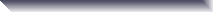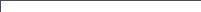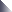SDL Component Suite - Math2The unit MATH2 offers some of the most fundamental procedures of univariate statistics (such as mean values, standard deviations, or the correlation coefficient for a series of data pairs). In addition, several more advanced mathematical methods (such as the calculation of eigenvectors and principal components, a simple clustering algorithm, and the smoothing of data) are contained in this unit. The unit MATH2 also contains a simple, yet powerful class (TCurveFit) which supports curve fitting of univariate data. More details can be found in the online help pages.

The unit MATH2 is part of the SDL Component Suite.

Please note that there are additional free programming examples which are not included in the component suite package:

 CLUSTER shows the usage of the clustering algorithm AgglomClustering SPLINE shows how to use cubic splines RANDGEN implements a configurable random generator

What's new:

• Release 10.7   [Aug-24, 2020]

• Release 10.6   [Mar-09, 2018]

• TCurveFit.CalcLinFit raises now an exception if the slope becomes infinite
• the function PolygonArea provides now a second overloaded version which counts the intersections
• the function DistanceToPolygon calculates the closest distance from a point to a polygon
• the function PolygonLength calculates the length of a polygon
• the functions TCurveFit.CalcPolyFit and TCurveFit.CalcCenteredPolyFit have been extended by alpha (the p-values of the parameters)

• Release 10.5   [Oct-10, 2016]

• Release 10.4   [Jun-02, 2015]

• the new function VertexCompAnalysis implements the vertex component analysis
• EnterStatValue is now a function returning the number of stored data points
• TMathExpression includes now a help context id on exceptions of the type ESDLMathExp.
• the function kMeansEstimatedSteps returns the estimated number of required processing steps of kMeans clustering
• the new procedure OuterRectOfPolygon returns the circumscribing rectangle of a polygon
• bug fix: PointIsInsidePolygon returned wrong result in degenerate cases

• Release 10.3   [Oct-06, 2014]

• speed of AgglomClustering increased substantially (factor five to ten)
• kMeansClustering now provides an overloaded version to control the selection of the initial centers
• kMeansClustering returns now the sum of squares of the intra-class distances
• the procedure TCurveFit.FlipXY exchanges the x and y variables
• TMathExpression now supports the predefined reference variable symbol ° (degree)
• bug fix: TCurveFit.SpearmanRankCorr now works with large data sets as expected (was round-off and overflow error)

• Release 10.2   [May-30, 2013]

• the new function MahalanobisDistance calculates the Mahalanobis distance between two points in n-dimensional space
• the new function MovingMedianSmooth smoothes data by a moving median
• the function EstimateByKNN now supports two new weighting modes (kwmLinear and kwmBiQuad)
• bug fix: TCurveFit.SpearmanRankCorr, TCurveFit.KendallsTau, and TCurveFit.KruskalGamma crashed if all data were constant
• bug fix: AgglomClustering returned slightly shifted distance values for Ward's method

• Release 10.1   [Oct-29, 2012]

• Release 10.0   [Oct-4, 2011]

• Release 9.7   [May-31, 2010]

• Release 9.51   [Dec-01, 2008]

• new function PointIsInsideConvexHull checks whether a point is inside or outside of a convex hull
• bug fix: procedure Convex2DHull produced wrong results if the x-coordinates of two neighboring points were exactly equal

• Release 9.5   [Oct-31, 2008]

• Release 9.0   [May-28, 2007]

• Release 8.5   [Feb-18, 2006]

• the routine CalcPrincComp is now available in a second overloaded version which allows to select whether to normalize the eigenvalues or not.
• CalcCovar now calculates the squared correlation coefficients and the sum of squared differences as additional options (mode=3, or 4)
• kMeansClustering implemented
• the coefficients of the Savitzky-Golay-Polynomials are now publicly available (see GolayPoly)
• TCurveFit.MeanAngleX, TCurveFit.MeanAngleY for the calculation of mean angles implemented

• Release 8.2   [Nov-03, 2004]

• Release 8.1   [Aug-29, 2004]

• Release 8.0   [Apr-15, 2004]

• new function PolygonArea implemented
• new function Convex2DHull calculates convex hull of a two-dimensional data set
• new class TMathExpression supports the interpretation of mathematical formulas
• new class TExtractor supports command driven extraction of values from strings
• new procedure to calculate penalized splines implemented (PenalizedCubicSpline)
• additional clustering method: Unweighted Pair Group with Arithmetic Mean (UPGMA)
• AgglomClustering has now different feedback mode
• TCurveFit: new properties MinX, MinY, MaxX, MaxY
• TCurveFit: ExportAsASC, ImportASC
• smoothed splines as additional curve fitting method (SplineSmoothingFactor, SmoothedSpline)

• Release 7.2   [Mar-23, 2003]

• MATH2 is now part of MathPack
• accuracy of SVD improved (had numerical problems when applying it to data covering a range of 8 decades or more)

• Release 7.0   [Sep-15, 2002]

• available for Delphi™ 7.0
• the unit is now CLX compatible
• bug fix: memory leak in CubicSpline fixed

• Release 6.5   [May-28, 2002]

• available for C++Builder™ 6.0
• SphereGreatCircleDist implemented

• Release 6.0   [Aug-06, 2001]

• available for Delphi™ 6.0
• CalcEigVec now increments the ProcStat variable with better granularity
• CalcCovar and CalcEigVec can be aborted by setting AbortMathProc
• EstimateByKNN implemented
• FindNearestNeighbors improved: distance calculation can now be performed by a user defined function

• Release 5.5  [May-21, 2000]

• available for C++Builder™ 5.0

• Release 5.0  [Oct-09, 1999]

• MATH2 is now available for Delphi™ 5.0 (as part of the SDL Component Suite 5.0)
• cubic spline interpolation implemented (methods and properties CubicSpline, SplineNatural1, SplineNaturalN, SplineDerivY1, SplineDerivYN)
• multiple linear regression implemented (MultiLinreg)
• singular value decomposition implemented (SingValDecomp, SingValEquSolve)
• bug fixed in MeanDistanceKNN and FindNearestNeighbors which caused
• wrong results with Delphi™ 1 to 3 (but not Delphi™ 4)

• Release 1.8  [Mar-27, 1999]

• MATH2 now available for C++Builder™ 4.0
• TCurveFit now additionally stores the data entered to it
• properties DataX and DataY implemented
• method RemoveStatValue implemented

• Release 1.7   [Jul-23, 1998]

• MATH2 now available for Delphi™ 4.0
• statistical function moved to unit STATIS (Kolmogorov-Smirnov, WeibullDensity, LnGamma, LnBeta, LnBinomCoeff, BinomCoeff, nDistri, tDistri)
• agglomerative clustering implemented

• Release 1.6 (Apr-08, 1998)

• MATH2 is now available for all versions of Delphi™ (1.0, 2.0, 3.0), and for C++Builder™ (1.0 and 3.0)
• DCommon no longer needed
• Kolmogorov-Smirnov one-sample test statistic
• GetEigenSize implemented
• WeibullDensity implemented
• PolyFit implemented (basic code supplied by Kerry L. Davison - thanks Kerry !)
• calculation of Gamma function
• calculation of Beta function
• calculation of binomial coefficient
• exception ESDLMath2Error introduced
• Polynomial smoothing of data series (alg. Savitzky/Golay)
• Smoothed first derivative of a series of data points

• Release 1.5 (May-28, 1997)
•
• MATH2 is now available for all three versions of Delphi™ and for C++Builder™